# 哈希表

## 概念

### 哈希表的基本思想

• 将记录的存储位置与它的关键字之间建立一个确定的关系H，使每个关键字和唯一的存储位置对应。而这种关系H就是该哈希表的一个哈希函数。
• 在查找时，只需要根据对应关系计算出给定的关键字值H(k)，就可以得到记录的存储位置。这样，不经过比较，一次存取就能得到所查元素的查找方法。

### 哈希表相关术语

• 哈希函数：在记录的关键字与记录的存储地址之间建立的一种对应关系。
• 冲突： 若关键字不同而函数值相同，则称这两个关键字为“同义词”，并称这种现象为冲突。
• 哈希查找：利用哈希函数进行查找的过程。
• 装填因子：记表中添入记录数为$m$，表长度为$n$，则装填因子为$\alpha = \frac{m}{n}$

### 哈希表性质

• 哈希表实际上是以空间换取时间，它的查找的时间效率一般比其它方法高，但消耗空间资源
• 冲突一般不可避免，发生冲突的次数与表的装填程度呈正相关
• 哈希函数相同的情况下，处理冲突的方法不同，所得哈希表的平均查找长度也不同
• 线性探测再散列处理冲突容易造成记录的“二次聚集”，即使得本不是同义词的关键字又产生新的冲突
• 对开放定址处理冲突的哈希表而言，表长必须≥记录数
• 链地址处理冲突的哈希表不要求表长必须≥记录数，它的平均查找长度主要取决于哈希函数本身

## 构造哈希函数

### 直接定址法

$$H(k) = ak+b$$

• 给定的一组关键字为关键字集合中全体元素，若不是全体关键字，则必有某地址单元空闲

### 数字分析法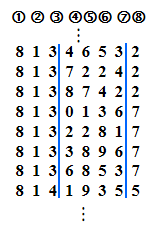• 关键字位数比哈希地址位数大，且可能出现的关键字事先知道的情况。

### 平方取中法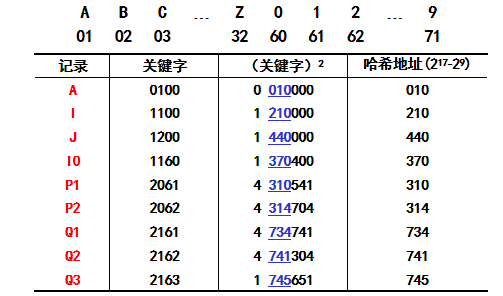• 适于不知道全部关键字情况
• 常用此法求取哈希函数

### 折叠法

• 移位叠加是将分割后的每一部分的最低位对齐，然后相加
• 间界叠加是从一端向另一端沿分割界来回折叠，然后对齐相加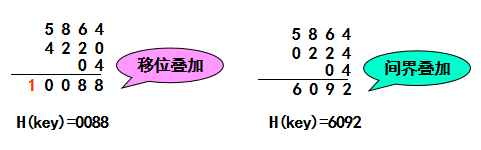• 适于关键字位数很多，且每一位上数字分布大致均匀情况

### 除数留余法

$$H(k) = k\mod{p}$$

### 随机数法

$$H(k) = random(k)$$

• 适于关键字长度不等的情况

## 解决冲突

### 开放定址法

$$H_i = (H(k)+d_i)\mod{m} \\i = 1,2,3,...,k\space(k\le m-1)$$

• 线性探测再散列：$d_i=1,2,...,m-1$
• 二次探测再散列：$k< \frac{m}{2}, \space d_i=1^{2},-1^{2},2^2,-2^2,...,\pm k^2$
• 伪随机探测再散列：$d_i$为伪随机数序列

#### 线性探测再散列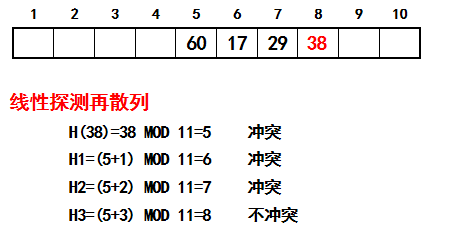#### 二次探测再散列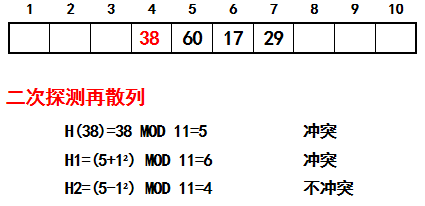#### 伪随机探测再散列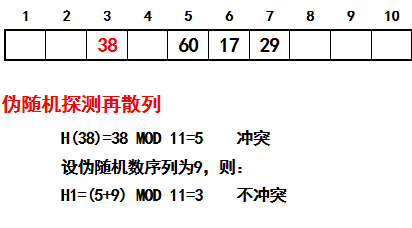## 平均找查长度ASL

### 平均找查长度

ASL（Average Search Length），即平均查找长度，在查找运算中，由于所费时间在关键字的比较上，所以把平均需要和待查找值比较的关键字次数称为平均查找长度

$$ASL = \sum_{i=1}^{n} p_ic_i$$

$$ASL=\frac{1}{n}\sum_{i=1}^{n} c_i$$

### 几种方法的ASL

#### 线性探测再散列

$$S_{nl} \approx \frac{1}{2}(1+\frac{1}{1- \alpha})$$

$$U_{nl} \approx \frac{1}{2}(1+ \frac{1}{(1-\alpha)^2})$$

#### 随机探测再散列、二次探测再散列和再哈希

$$S_{nr} \approx -\frac{1}{\alpha}ln(1-\alpha)$$

$$U_{nr} \approx \frac{1}{1-\alpha}$$

#### 链地址

$$S_{nc} \approx 1+\frac{\alpha}{2}$$

$$U_{nc} \approx \alpha + e^{-\alpha}$$